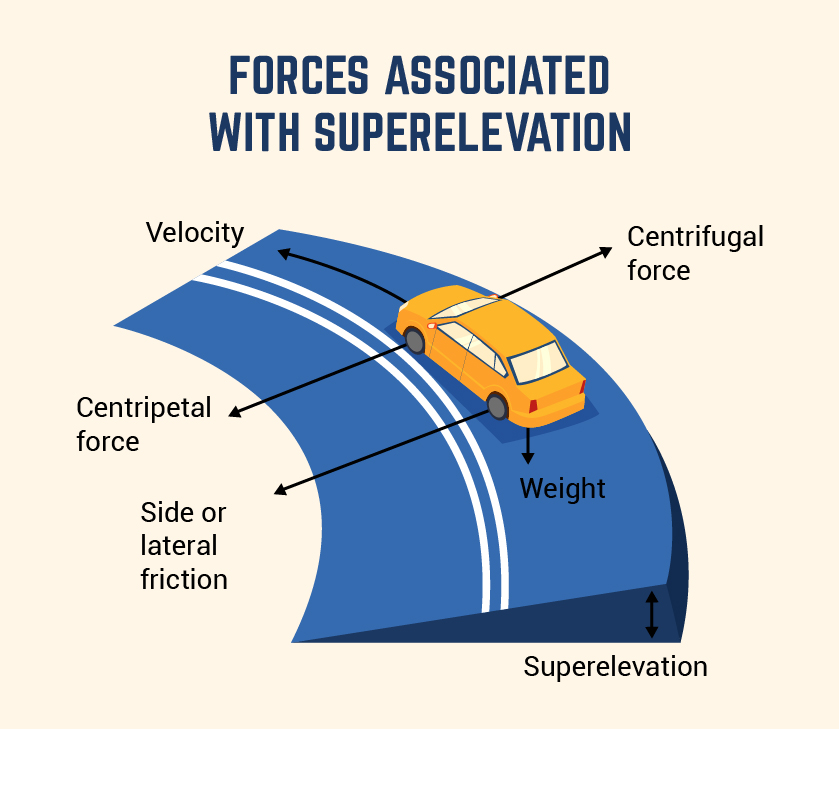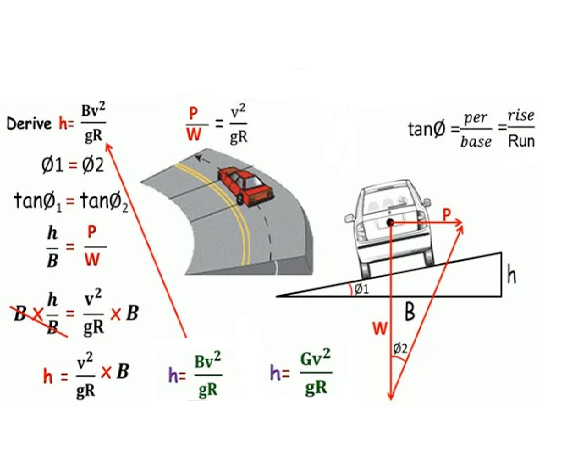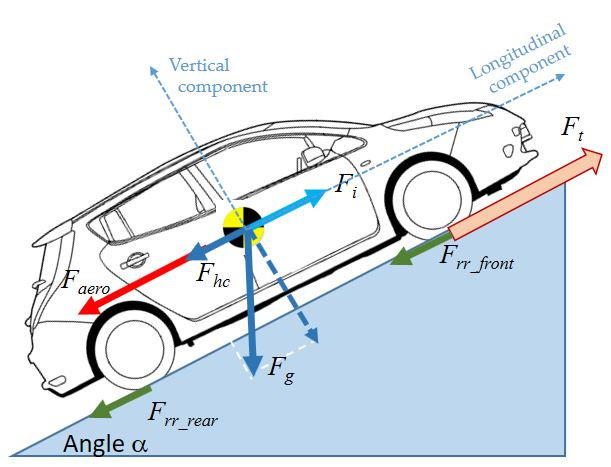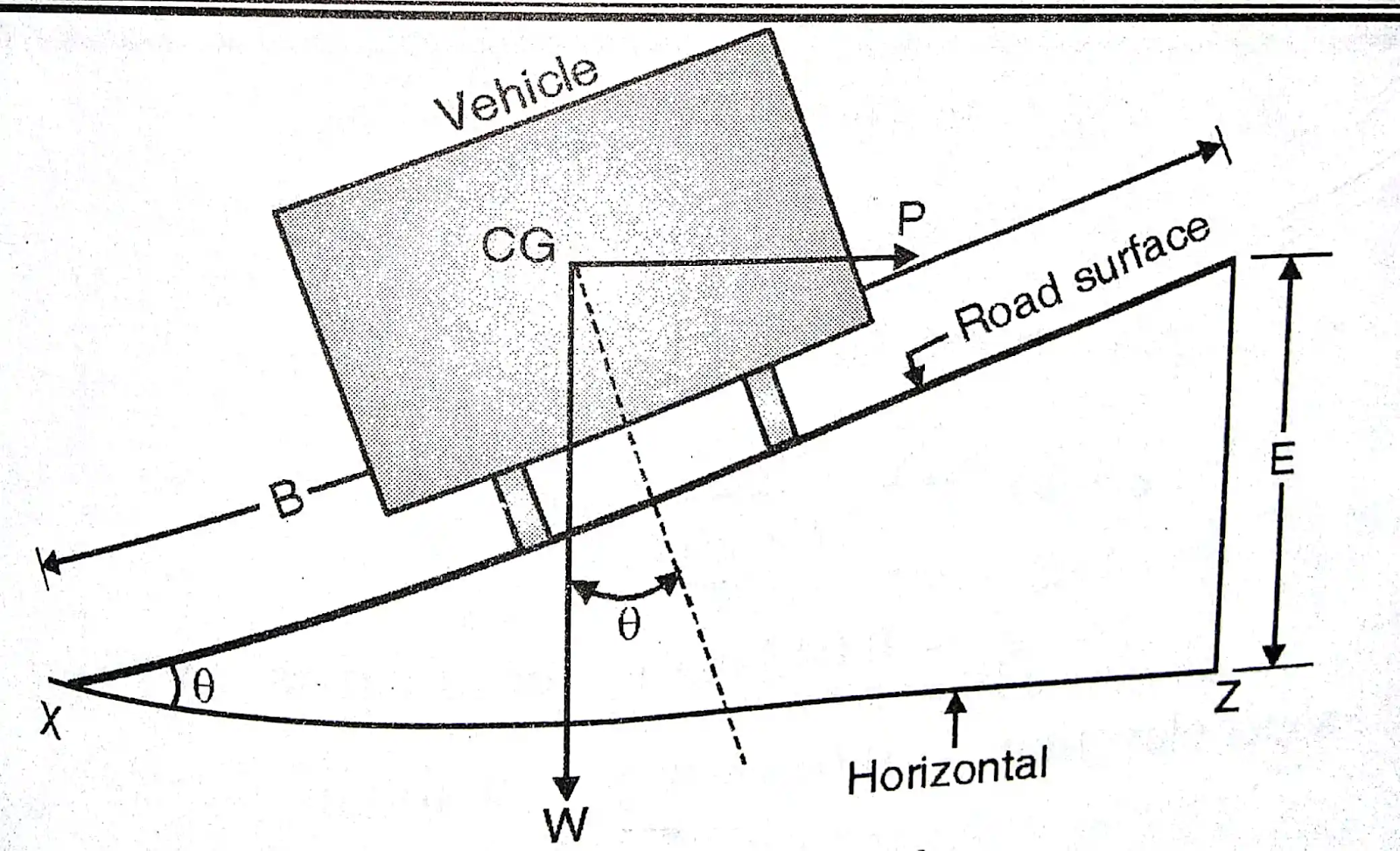### Superelevation

• Superelevation

1. In order to counteract the effect of centrifugal force & to reduce the tendency of the vehicle to overturn & skid, the outer edge of pavement is raised with respect to the inner edge thus providing a transverse slope throughout the length of the horizontal curve.

2. The transverse inclination to the pavement surface is known as “SUPERELEVATION / CANT / BANKING”

3. If e is the superelevation rate & E is total super elevation

With respect to rise in outer edge of pavement with respect to inner edgeSuperelevation on the horizontal curve is one of the most important features in the construction of the roads. It is very essential to provide superelevation in roads for the safe movement of vehicles on the curved portion of the roads.

Consider a vehicle moving on the circular horizontal curve of the road.

The speed of the moving vehicle on the circular path is V m/s

The curve of the radius is R meters

The forces which are acting on a vehicle while  it is moving on the horizontal circular curve are as follows.

The centrifugal force which is acting horizontally out work through the center of gravity, P=( W V2/gR)

The weight(W) of the vehicle which is acting vertically downwards through the center of gravity.

The friction which is developed between the wheel of the vehicle and the pavement acting transversely along the pavement surface towards the center of the curve.

The centrifugal force is opposed by the corresponding value of the friction developed and by a component of the force of gravity due to the Superelevation is provided.

SuperElevation / Banking of road reduces the effect of centrifugal force on the running wheels. If superelevation is not provided with the entire centripetal force is produced by the friction between the vehicle’s tires and the roadway, thus results in reducing the speed of a vehicle.

1. Super elevation is provided to achieve the higher speed of vehicles.
It increases the stability of fast-moving vehicles when they pass through a horizontal curve, and it also decreases the stresses on the foundation.

2. In the absence of super elevation on the road along curves, potholes are likely to occur at the outer edge of the road.

3. The Indian road congress(IRC) has prescribed the max value of Super Elevation is 1 in 15.

Derivation of Super Elevation :

As per the figure, the below forces are acting on a carIn order to find out the angle of elevation (Super Elevation) the “tan” formula is used

From above fig, tanθ  = Opposite Side⁄Adjacent Side

therefore,               tanθ  = E⁄B

The below forces are acting on the vehicle as mentioned in figure:

Weight of the vehicle  = W kg () ;

Centripetal force = P (→);

Frictional forces   = F1 & F2 (← );

You can check out the below figure for more ideas.Hence, P.Cosθ  = W.Sinθ+F1+F2

where              F = fR

P.Cosθ = WSinθ + fR1 + fR2

= W.Sinθ + f(R1 + R2)

= W.Sinθ + f(PSinθ+WCosθ)

P.Cosθ-f.PSinθ = W.Sinθ+f.WCosθ

Divide with “W.Cosθ”;

(P.Cosθ-f.PSinθ)/W.Cosθ  = (W.Sinθ+f.WCosθ)⁄W.Cosθ

P/W -(f.P/W)tanθ = tanθ + f

P/W (1-f.tanθ) = tanθ+f

P/W = (tanθ+f)/1-f.tanθ

P/W = e + f

But, P/W = V2/ gR

Therefore, e + f = V2/ gR

Where,

e = rate of Superelevation in %

V = velocity of vehicle in m/s

f = lateral friction factor = 0.15

g = acceleration due to gravity = 9.81 m/s2

R = radius of circular curve in meters.

If velocity is in KMPH then  e + f = V2/ 127R